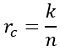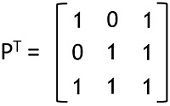# Linear Block Code

Linear block code is a type of error-correcting code in which the actual information bits are linearly combined with the parity check bits so as to generate a linear codeword that is transmitted through the channel. Another major type of error-correcting code is convolution code.

In the linear block code technique, the complete message is divided into blocks and these blocks are combined with redundant bits so as to deal with error detection and correction.

## Content: Linear Block Code

### What is Error Correcting Code?

While discussing interleaving, we have mentioned that the bursts of errors are redistributed over codewords (the process is named as interleaving) after which error-correcting codes can be applied in order to correct the errors.

Now, the question arises of what exactly error correction refers to and why it is needed.

Whenever we deal with data communication, then one of the major concerns associated with the transmission of the data is the introduction of errors. These errors must be removed as the presence of errors changes the actual information content. The sole purpose of applying error correction codes is to facilitate the detection and even correction of the data which is transmitted over communication channel by introducing redundancies within the actual message bits.

The error-correcting codes are nothing but the addition of redundancies (unnecessary bits) to the actual message bits so that the receiver must properly decode the actual message signal from the encoded one.

It is to be noted here that the error-correcting codes are applicable to digital signals and not analog ones. More simply, the signals that are in the form of 1s and 0s i.e., the binary form.

There are two techniques for error control coding, namely

• Linear Block Coding
• Convolution Coding

We already know that encoding enables the addition of coding bits to the data stream, done at the transmitter side. While decoding is the recovery of the actual data stream from the coded one, done at the receiver end. However, the codec performs the action of both coding and decoding.

## Linear Block Coding

In block coding, the complete message bits are divided into blocks where each block holds the same number of bits. Suppose each block contains k bits, and each k bits of a block defines a dataword. Hence, the overall datawords will be 2k. At this particular point, we have not considered any redundancies, thus, we only have the actual message bitstream converted into datawords.

Now, in order to perform encoding, the datawords are encoded as codewords having n number of bits. We have recently discussed that a block has k bits and after encoding there will be n bits in each block (of course, n>k) and these n bits will be transmitted across the channel. While the additional n-k bits are not the message bits as these are named as parity bits but during transmission, the parity bits act as they are a part of message bits.

So, structurally, a codeword is represented as:Hence, the possible codewords will be 2n out of which 2k contains datawords. During transmission, if errors are introduced then most probably, the permissible codewords will be changed into redundant words which can be detected as an error by the receiver.

In reference to the terms, codewords and datawords, a term code rate is used which is defined as the ratio of dataword bits to the codeword bits. Thus, is represented as:A code is represented as (n,k). Consider an example where n=6 and k=3 then code will be (6,3), indicating that a dataword of 3 bits is changed into a codeword of 6 bits.

### Explanation with Example

Consider a repetition code, to understand the general properties of block code. In a repetition code, each individual bit acts as a dataword. Thus, k = 1 and n-redundancy encoding will provide n-bits at the output of the encoder.

If n = 3, then for binary value 1 at the input of encoder, the output codeword will be 111. While for binary value 0, the output codeword will be 000. The logic circuit within the decoder decodes codeword 111 as 1 while codeword 000 as 0. This is achieved through proper synchronization between the encoder and the decoder.

However, for this particular case, if at the input of the decoder, codeword other than 111 or 000 is achieved then the decoder will detect error full transmission and will send an automatic repeat request for retransmission of the codeword. Sometimes to deal with a single error issue, forward error correction is used that produces the output according to the majority vote.

This means that if at the input of the decoder, the obtained codeword has either two or three 1’s (such as 111, 110, 101, 011) then it will give 1 as the output. However, in the converse case, if the codeword has two or three 0’s (such as 001, 010, 100, 000) then the output will be 0.

Sometimes due to noise, the codeword gets hampered by not only a single error but also by 2 or even 3 successive errors. Like 111 can be changed into 000 or vice versa. In this case, from the above-discussed method, the obtained output will also be an error and not the actual information. But the chance of occurrence of 2 or 3 simultaneous errors is very less.

Thus, it is said that a code is said to be linear if two linearly combined codewords produce another codeword.

Suppose for k = 3, the possible datawords i.e., 23 = 8 are converted into codewords by performing modulo-2 addition with parity bits.

DatawordParity BitCodeword
00000000
00110011
01010101
01100110
10011001
10101010
11001100
11111111

Here the assigned parity bits offer even parity to the datawords, this means the total 1’s in the codewords after addition will be even. An even parity provides codeword modulo sum to zero. As it is clear from the above table the addition of each bit of individual codeword is 0 (as 0+0 is 0, 1+1 is 0, 1+0 is 1 and 0+1 is 1).

For two codewords, hamming distance corresponds to the total number of variations in the positions of various bits between two of them.

Suppose we have 0011 and 0010, then here the two codewords are different at a single position only thus, the hamming distance is one.

When we talk about minimum hamming distance or minimum distance, then it is related to the smallest hamming distance existing between two codes. For any codeword, the minimum distance is evaluated according to the total binary 1’s in it. For codeword 0011 the minimum distance will be two.

### Linear Encoding of Block Codes

Now, consider matrices to understand more about linear block codes.

Suppose, we have a dataword, 101, represented by row vector d.

d = 

The codeword for this with the even parity approach discussed above will be 1010, given by row vector c.

c = 

Generally, generator matrix, G is used to produce codeword from dataword. The relation between c, d and G is given as:

c = dG

For a code (6,3), there will be 6 bits in codeword and 3 in the dataword. In a systematic code, the most common arrangement has dataword at the beginning of the codeword. To get this, identity submatrix is used in conjunction with parity submatrix and using the relation d.G, we can haveIt is clearly shown that dataword is present as the first 3 bits of the obtained codeword while the rest 3 are the parity bits.

### Linear Decoding of Block Codes

To decode the actual dataword from the obtained codeword at the receiver, transpose of parity matrix is done.Further, the parity check matrix, H is obtained by the combination of transpose of parity matrix and the identity matrix.For the matrix H, the decoder can analyze the parity bits from the obtained codewords. Here, the total number of rows in the above matrix represents the number of parity bits i.e., n-k while the number of columns shows the number of bits in the codeword i.e., n. In this particular example the number of rows is 3, representing total 3 parity bits and the number of columns here is 6 showing n i.e., the total bits in the codeword.

A fundamental property of code matrices states that,

GHT = 0

For a received codeword the verification of correction is obtained by multiplying the code with HT.

cHT = 0

As we know that,

c =dG

So, substituting dG in replace of c in second last equation, we will have,

dGHT = 0

If this product is unequal to 0 then this shows the presence of error. Generally, s called syndrome is given as:

s = cHT

A relation between transmitted and received codeword is written in a way that,

cR = cT + e

This means that the received codeword must be necessarily equal to the summation of the actually transmitted codeword and the error vector.